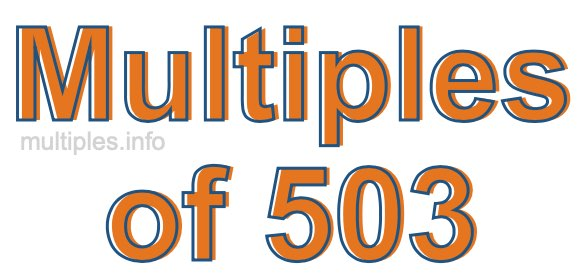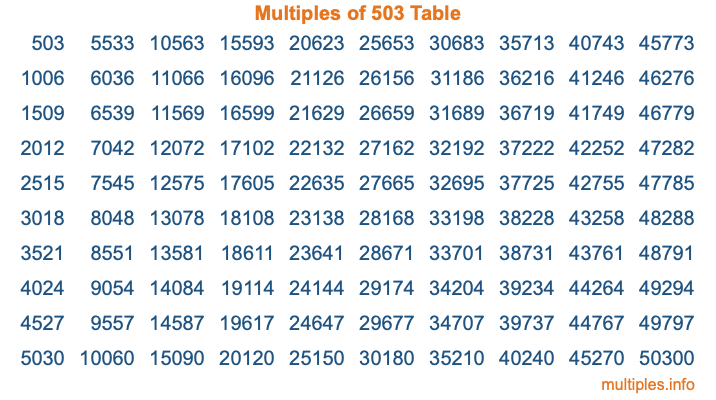Multiples of 503Welcome to the Multiples of 503 page. Here we will first teach you everything you will ever need to know about the multiples of 503, and then give you a study guide summary of everything we taught you to make sure you remember it all. Use this page to look up facts and learn information about the multiples of 503. This page will make you a multiples of five hundred three expert!

Definition of Multiples of 503
Multiples of 503 are all the numbers that when divided by 503 equal an integer. Each of the multiples of 503 are called a multiple. A multiple of 503 is created by multiplying 503 by an integer.

Therefore, to create a list of multiples of 503, you start with 1 multiplied by 503, then 2 multiplied by 503, then 3 multiplied by 503, and so on for as long as you want. Thus, the list of the first five multiples of 503 is 503, 1006, 1509, 2012, and 2515. To see a larger list of multiples of 503, see the printable image of Multiples of 503 further down on this page. We also have a category where you can choose any nth multiple of 503.

Multiples of 503 Checker
The Multiples of 503 Checker below checks to see if any number of your choice is a multiple of 503. In other words, it checks to see if there is any number (integer) that when multiplied by 503 will equal your number. To do that, we divide your number by 503. If the the quotient is an integer, then your number is a multiple of 503.

Is  a multiple of 503?

Least Common Multiple of 503 and ...
A Least Common Multiple (LCM) is the lowest multiple that two or more numbers have in common. This is also called the smallest common multiple or lowest common multiple and is useful to know when you are adding our subtracting fractions. Enter one or more numbers below (503 is already entered) to find the LCM.

Check out our LCM Calculator if you need more details about the Least Common Multiple or if you need the LCM for different numbers for adding and subtraction fractions.

nth Multiple of 503
As we stated above, 503 is the first multiple of 503, 1006 is the second multiple of 503, 1509 is the third multiple of 503, and so on. Enter a number below to find the nth multiple of 503.

th multiple of 503

Multiples of 503 vs Factors of 503
503 is a multiple of 503 and a factor of 503, but that is where the similarities end. All postive multiples of 503 are 503 or greater than 503. All positive factors of 503 are 503 or less than 503.

Below is the beginning list of multiples of 503 and the factors of 503 so you can compare:

Multiples of 503: 503, 1006, 1509, 2012, 2515, etc.

Factors of 503: 1, 503

As you can see, the multiples of 503 are all the numbers that you can divide by 503 to get a whole number. The factors of 503, on the other hand, are all the whole numbers that you can multiply by another whole number to get 503.

It's also interesting to note that if a number (x) is a factor of 503, then 503 will also be a multiple of that number (x).

Multiples of 503 vs Divisors of 503
The divisors of 503 are all the integers that 503 can be divided by evenly. Below is a list of the divisors of 503.

Divisors of 503: 1, 503

The interesting thing to note here is that if you take any multiple of 503 and divide it by a divisor of 503, you will see that the quotient is an integer.

Multiples of 503 Table
Below is an image of the first 100 multiples of 503 in a table. The table is in chronological order, column by column. The first column has the first ten multiples of 503, the second column has the next ten multiples of 503, and so on.The Multiples of 503 Table is also referred to as the 503 Times Table or Times Table of 503. You are welcome to print out our table for your studies.

Negative Multiples of 503
Although not often discussed or needed in math, it is worth mentioning that you can make a list of negative multiples of 503 by multiplying 503 by -1, then by -2, then by -3, and so on, to get the following list of negative multiples of 503:

-503, -1006, -1509, -2012, -2515, etc.

Multiples of 503 Summary
Below is a summary of important Multiples of 503 facts that we have discussed on this page. To retain the knowledge on this page, we recommend that you read through the summary and explain to yourself or a study partner why they hold true.

There are an infinite number of multiples of 503.

A multiple of 503 divided by 503 will equal a whole number.

503 divided by a factor of 503 equals a divisor of 503.

The nth multiple of 503 is n times 503.

The largest factor of 503 is equal to the first positive multiple of 503.

503 is a multiple of every factor of 503.

503 is a multiple of 503.

A multiple of 503 divided by a divisor of 503 equals an integer.

503 divided by a divisor of 503 equals a factor of 503.

Any integer times 503 will equal a multiple of 503.

Multiples of a Number
Here you can get the multiples of another number, all with the same attention to detail as we did for multiples of 503 on this page.

Multiples of
Multiples of 504
Did you find our page about multiples of five hundred three educational? Do you want more knowledge? Check out the multiples of the next number on our list!